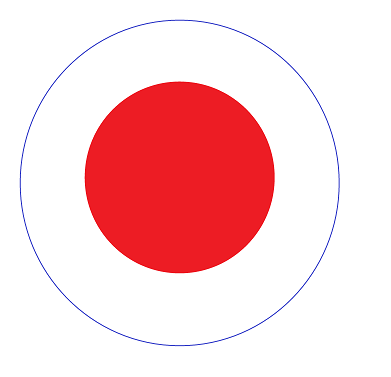# Calculate a random point inside a circle,How to random calculate a random point inside a circle

I’m have drawn a circle in my 3d world, now I want to calculate a random point inside this circle. But not in the while circle but in a certain area inside this circle.The random point should be inside the red area (radius divided by 2)

Uhm just use Random.insideUnitCircle and multiply by your desired radius and you get a random vector which length is between 0 and your desired radius. Of course the origin is at the center of the circle. If you want the center somewhere else, just add that vector to your desired center

``````Vector2 randomPoint = centerPoint + Random.insideUnitCircle * radius * 0.5f;
``````

Note that `* 0.5f` does cover your requirement of “half the radius”.

ps: You said in your “3d world” however a circle is just a 2d shape. You can use 2d shapes in a 3d world however there are endless ways how such a circle could be oriented in 3d space. If you’re actually not looking for a circle but a sphere, just use Random.insideUnitSphere instead.